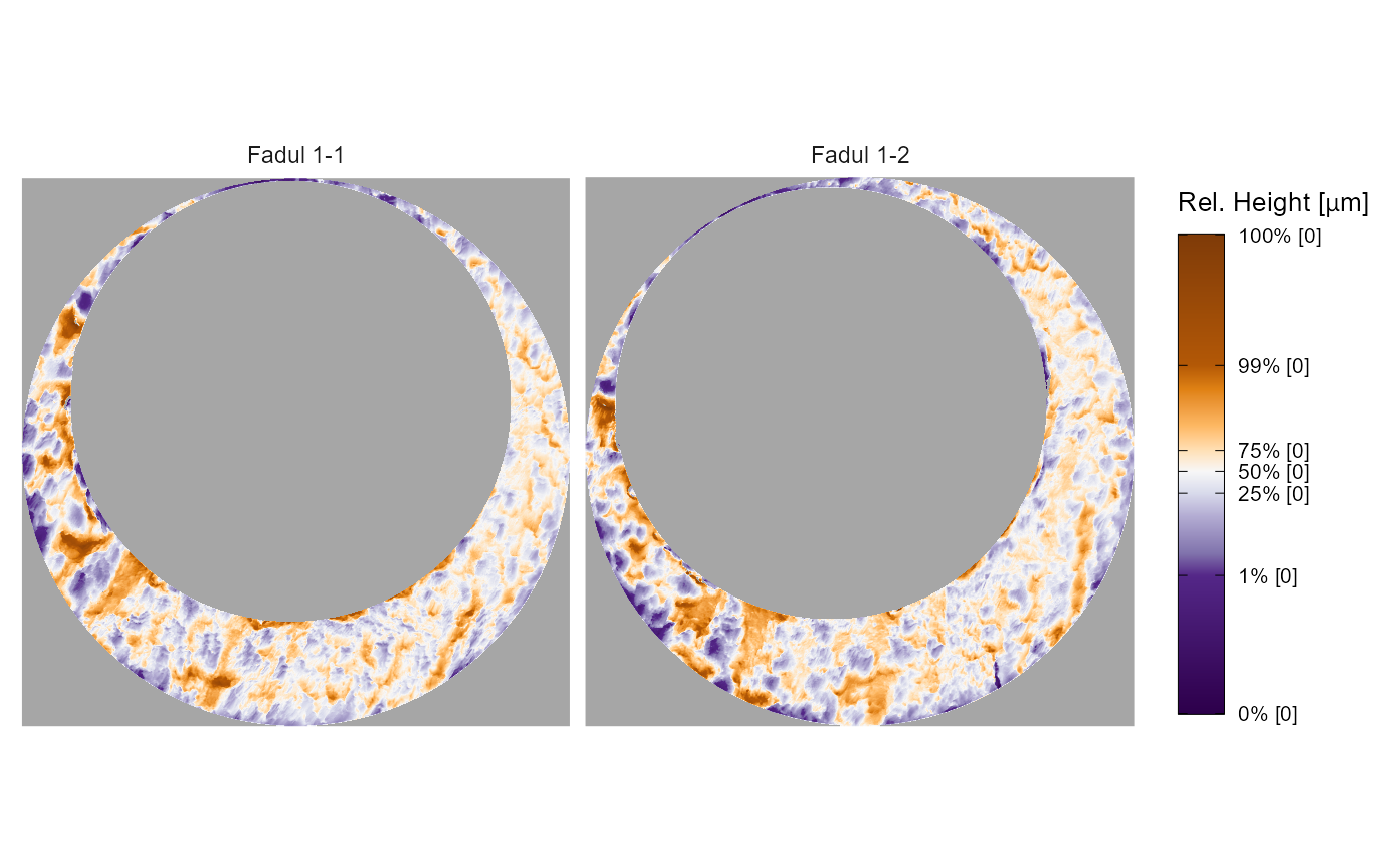Plots the surface matrices in a list of x3p objects. Either creates one plot faceted by surface matrix or creates individual plots per surface matrix and returns them in a list.

x3pListPlot(
x3pList,
type = "faceted",
legend.quantiles = c(0, 0.01, 0.25, 0.5, 0.75, 0.99, 1),
height.quantiles = c(0, 0.01, 0.025, 0.1, 0.25, 0.5, 0.75, 0.9, 0.975, 0.99, 1),
height.colors = rev(c("#7f3b08", "#b35806", "#e08214", "#fdb863", "#fee0b6",
"#f7f7f7", "#d8daeb", "#b2abd2", "#8073ac", "#542788", "#2d004b")),
na.value = "gray65"
)

## Arguments

x3pList

a list of x3p objects. If the x3p objects are named in the list, then these names will be included in the title of their respective plot

type

dictates whether one plot faceted by surface matrix or a list of plots per surface matrix is returned. The faceted plot will have a consistent height scale across all surface matrices.

legend.quantiles

vector of quantiles to be shown as tick marks on legend plot

height.quantiles

vector of quantiles associated with each color defined in the height.colors argument

height.colors

vector of colors to be passed to scale_fill_gradientn that dictates the height value colorscale

na.value

color to be used for NA values (passed to scale_fill_gradientn)

## Value

A ggplot object or list of ggplot objects showing the surface matrix height values.

## Examples

data(fadul1.1_processed,fadul1.2_processed)